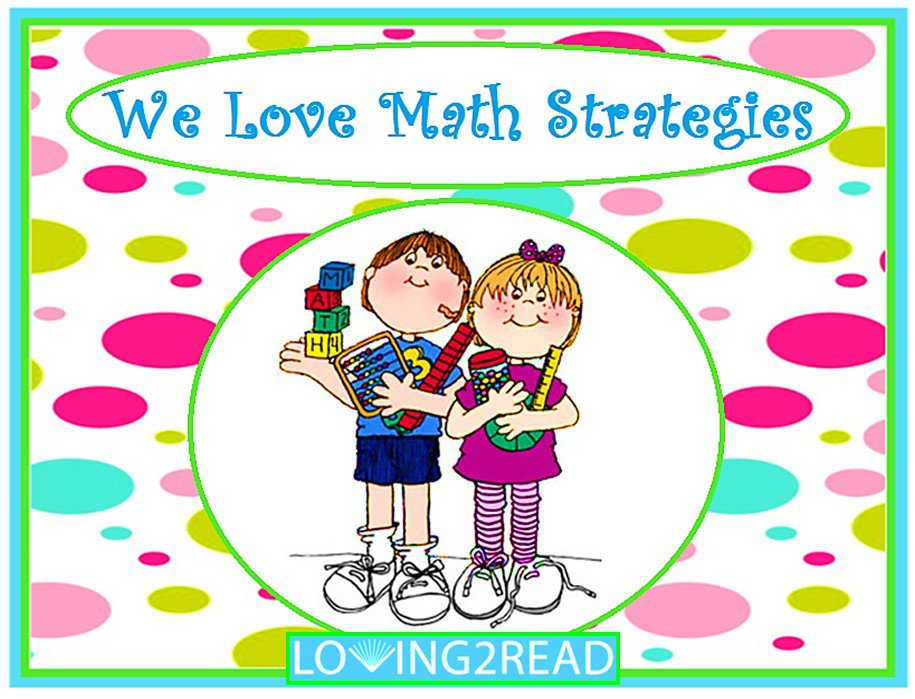# We Love Math Strategies

## Book information

Math strategies will help you master math! Enjoy reading this math book over and over again, math fluency is the key. Loving2Read...Where Wild & Crazy Kids Read and Succeed!

Concepts and Skills:

• math
• math strategies
• math manipulatives
• drawing pictures
• math doubles
• counting on
• math vocabulary
• mental math
• number paths
• math notebook